# Java算法入门以及常见时间复杂度的推导

「这是我参与11月更文挑战的第11天，活动详情查看：2021最后一次更文挑战」。

# 3 算法时间复杂度

## 3.2 大O的推导

1. 用常数1取代运行时间中的所有加法常数。
2. 在修改后的运行次数函数中，只保留最高阶项。
3. 如果最高阶项存在且不是1，则去除与这个项相乘的常数。
4. 考虑n 变大的情况。去除其他影响较小的部分，保留最大的部分。

## 3.3 常见时间复杂度介绍

1. O(1)，也叫常数时间，例如通过索引查找数组元素。
2. O(log n)，也叫对数时间，这样的算法包括二分查找。
3. O(n)，也叫线性时间，这样的算法包括简单查找。
4. O(n log n)，也叫线性对数时间，这样的算法包括快速排序。
5. O(n²)，也叫平方时间，这样的算法包括选择排序。
6. O(n³)，也叫立方时间，不常见。
7. O(2^n^)，也叫指数时间，不常见。
8. O(n!)，也叫阶乘时间，这样的算法包括旅行商问题的解决方案，包括写出1~n的所有全排列，不常见。
9. O(n^n^)，也叫完全平方时间，时间复杂度最高，不常见。

O(1)<O(logn)<O(n)<O(nlogn)<O(n^2^)<O(n^3^)<O(2^n^ ) <O(n!)<O(n^n^)

### 3.3.1 O(1)

``````/**
* 案例1:求1+2+3+4+……+n的和
* 使用高斯算法,无论n为多少,只需要下面一个步骤就能求出和,算法的时间复杂度为O(1)
*/
private static long O1(int n) {
long sum=(1 + n) * n / 2;
return sum;
}

### 3.3.2 O(n)

``````/**
* 案例2:求1+2+3+4+……+n的和
* 使用传统循环相加算法,n为多少,需要循环多少次,排除
* 算法的时间复杂度为O(n),线性时间,算法效率明显要低于高斯算法
*/
private static long O2(int n) {
long sum = 0;
// n为多少,这里就需要循环多少次
for (int i = 1; i <= n; i++) {
sum += i;
}
return sum;
}

### 3.3.3 O(logn)

``````/**
* 案例3:对于2的x次方值n,求x的值是多少
* 采用下面的循环算法，循环逼近.
* 该算法可以换算为，有多少个2相乘后等于n，则会退出循环。由2^x=n 得到 x=log2n。
* 这个x就是时间复杂度，所以这个算法的时间复杂度为O(logn)。
*/
private static long O3(int n) {
long m = 1;
long x = 0;
while (m == n) {
//下面两句话看成一段时间复杂度为O(1)的循环程序步骤，需要执行logn次
x++;
m *= 2;
}
return x;
}

### 3.3.4 O(n²)

``````/**
* 案例4: O(n^2)
* 输入为n时，需要执行n^2次内层的计算
*/
private static long O4(int n) {
long sum = 0;
long n2 = n ^ 2;
for (int i = 0; i < n2; i++) {
/*时间复杂度为O(1)的程序步骤*/
sum += i;
}
return sum;
}

## 3.4 复杂语句的时间复杂度推导

### 3.4.1 多重循环

``````private static void O5(int n) {
for (int i = 0; i < n; i++) {
for (int j = i; j < n; j++) {
/* 时间复杂度为O(1)的程序步骤序列 */
}
}
}

### 3.4.2 顺序语句

``````private static long O6(int n) {
//1次
long sum = 0;
//n次
for (int i = 0; i < n; i++) {
/* 时间复杂度为O(1)的程序步骤序列 */
}
//n(n+1)/2次
for (int i = 0; i < n; i++) {
for (int j = i; j < n; j++) {
/* 时间复杂度为O(1)的程序步骤序列 */
}
}
//1次
return sum;
}

### 3.4.3 条件判断语句

``````private static void O7(int n) {
if (n == 1) {
//n次
for (int i = 0; i < n; i++) {
/* 时间复杂度为O(1)的程序步骤序列 */
}
} else {
//n(n+1)/2次
for (int i = 0; i < n; i++) {
for (int j = i; j < n; j++) {
/* 时间复杂度为O(1)的程序步骤序列 */
}
}
}
}

### 3.4.4 递归

``````private static long O8(int n) {
if (n <= 1) {
return 1;
} else {
return O8(n - 1) + O8(n - 2);
}
}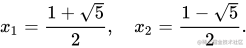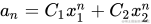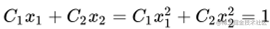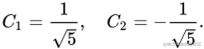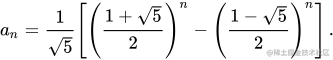1. 求复杂的时间复杂度需要大学数学的知识了；
2. 代码中慎用递归。

1. 《大话数据结构》
2. 《算法图解》
3. 《我的第一本算法书》